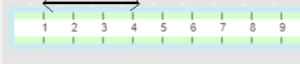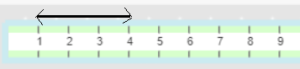# Math in Focus Grade 2 Chapter 13 Practice 3 Answer Key Measuring in Inches

This handy Math in Focus Grade 2 Workbook Answer Key Chapter 13 Practice 3 Measuring in Inches detailed solutions for the textbook questions.

## Math in Focus Grade 2 Chapter 13 Practice 3 Answer Key Measuring in Inches

Question 1.
Check (✓) the correct way to measure the length of the pencil.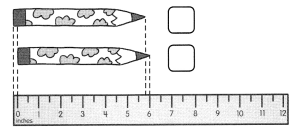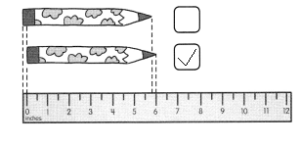Use an inch ruler to measure each part of a line. Then answer the questions.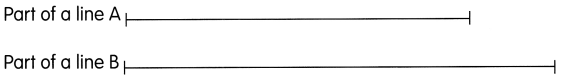Question 2.
How long is Part of a line A? ___________ in.

Part of line A is 3 in.

Question 3.
How long is Part of a line B? ___________ in.

Part of line B is 4 in.

Use a string and an inch ruler to measure each curve.

Question 4.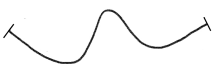___________ in.

The above cure is 3 in.

Question 5.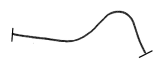___________ in.

The above figure is 2 in.

Fill in the blanks.

Question 6.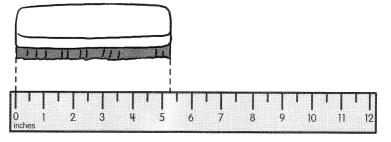The eraser is about ___________ inches long.

The eraser is about 5  inches long.

Question 7.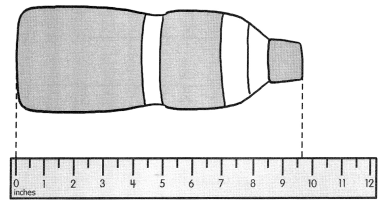The bottle is about ___________ inches long.

The bottle is about 10 inches long.

Question 8.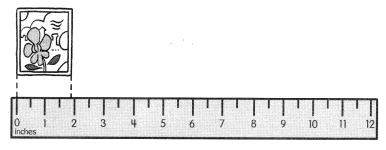The sticker is about ___________ inches long.

The sticker is about 2 inches long.

Fill in the blanks.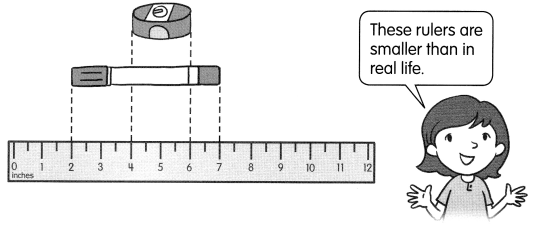Question 9.
The marker is ___________ inches long.

The marker is 5 inches ( 7 – 2 = 5 )  long.

Question 10.
The pencil sharpener is _________ inches long.

The pencil sharpener is 2 inches ( 6 – 4 =2 ) long.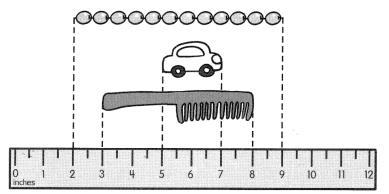Question 11.
The length of the comb is ____________ inches.

The length of the comb is 5 inches ( 8 – 3 = 5 ).

Question 12.
The length of the string of beads is ____________ inches.

The length of the string of beads is 7 inches ( 9 – 2 = 7 ).

Question 13.
The length of the toy car is ____________ inches.

The length of the toy car is 2 inches ( 7 – 5 = 2 ).

Use your inch ruler to draw.

Question 14.
Part of a line A that is 4 inches long.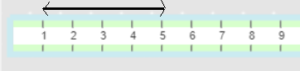Question 15.
Part of a line B that is 2 inches long.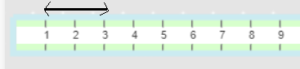Question 16.
Part of a line C that is 5 inches long.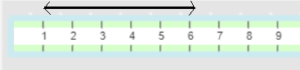Question 17.
Part of a line D that is 1 inch long.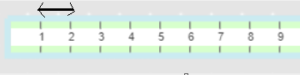Question 18.
Part of a line E that is 2 inches shorter than Part of a line C.